• jquery标签选择器
千次阅读
2019-09-23 22:25:36

## 1. 基本选择器

//标签
$("div").css("background","red"); //id$("#active").css("background","orange");

//类
$(".box").css("background","green"); //群组$(".box,#active").css("width",200);

//jq里面没有优先级，只有先后顺序


## 2. 层次选择器

//1. 后代选择器 所有的后代
$("div span").css("font-size",20); //2. 直接后代选择器$("div>span").css("color","red");
//3.相邻兄弟选择器：紧跟在后面的一个
$("h2+ul").css("background","pink"); //4.后面所有的兄弟选择器$("h2~ul").css("background","red");


jquery里也有this

$("h2").click(function(){$(this).next("ul").css("background","Red"); //紧跟在后面的一个
$(this).nextAll("ul").css("background","Red"); //后面所有的兄弟$(this).prev("ul").css("background","red"); //上一个兄弟
$(this).prevAll("ul").css("background","red"); //上面所有的兄弟$(this).siblings("ul").css("background","red");//所有的兄弟 ul 元素
})


## 3.基本过滤选择器

//:first :第一个子元素
$("ul li:first").css("background","pink"); //:last :最后一个子元素$("ul li:last").css("background","pink");

//:first-child 第一个子元素
$("ul :first-child").css("background","red"); //:eq(下标)：获取对应下标的元素$("p:eq(2)").css("color","red");

//:even 偶数 :odd
$("li:even").css("background","red");$("li:odd").css("background","pink");

//:gt(下标)：获取下标大于某个数的元素   lt
$("li:lt(3)").css("background","red");$("li:gt(2)").css("background","gray");

//:not(选择器) ：除了
$("li").not("#box").css("background","orange");  ## 4. 内容过滤选择器 //:contains(内容) 匹配包含特定内容的标签$("li:contains(内容)").css("color","red");

//:has(选择器) 匹配包含特定标签的标签
$("li:has(span)").css("color","red"); //empty 匹配空标签 任何东西都没有 //$(":empty").remove();

//:parent 获取有子元素的li标签
$("li:parent").remove();  ## 5.属性选择器 //有type类型的input标签$("input[type]");
$("input[type=radio]"); //有type类型的并且值 不为radio input标签$("input[type!=radio]");
//有type类型的并且值  以开头 input标签
console.log($("input[type^=r]")); //有type类型的并且值以 结尾 input标签 console.log($("input[type$=x]")); //有type类型的并且值包含某个值 input标签 console.log($("input[type*=o]"));


## 6.表单相关选择器

//1.快速获取表单元素
console.log($(":input")); //获取到所有表单元素 console.log($(":radio")); //获取radio类型的元素
console.log($(":text")); //获取text类型的元素 console.log($("checkbox")); //获取checkbox类型的元素

//2. enabled:可用 disable：禁用
$(":disabled").css("background","red"); //3.visible：显示 hidden：隐藏 console.log($("input:hidden"));

//4.checked:获取选择的input标签
console.log($(":radio:checked"));  ## 7.循环 ### 1.map $.map : 有返回值，返回值会组成一个新的数组
//语法：$.map(循环的对象,function(value,index){}) //语法：$(选择器).map(function(value,index){})


### 2.each

$.each:没有返回值 语法：$.each(循环的对象,function(index,value){})
语法：$(选择器).each(function(index,value){})  更多相关内容 • ## JQuery标签选择器 千次阅读 2020-07-12 23:22:31 3、标签选择器 语法：$(" 标签名 ") 根据给定的元素名匹配所有元素 4、通配符选择器 语法：$(" * ") 匹配所有元素 5、并集选择器 语法：$(" selector1,selector2,selectorN ") 将每一个选择器匹配到的元素合并...

#### 一、基本选择器

根据给定的类匹配元素。

匹配所有元素

语法：$(" selector1,selector2,selectorN ") 将每一个选择器匹配到的元素合并后一起返回。 ##### 6、交集选择器 语法：$(" selector1selector2 ")

注意选择器1和选择器2之间没有空格，注意区分后代选择器。

#### 二、层级选择器

语法：：$(" E F ") 说明：E与F之间用空格分开，注意：E必须包含F 功能描述：在给定的祖先元素下匹配所有的后代元素（不一定是直系 ##### 2、子选择器 语法：：$(" E> F ")

说明：E必须包含F

功能描述：选择匹配的F元素,F必须是E的直系子元素

##### 3、相邻兄弟选择器

语法：：$("E+ F ") 等价于$(“E”).next(“F”)

说明：E,F元有一个相同的父级元素，换句话说E和F是同辈元素

功能描述：选择匹配的F元素,注意E和F必须相邻且F在E的下面

##### 4、通用兄弟选择器**

语法：：$("E~ F ") 等价于$(“E”).nextAll(“F”)

说明：E,F元有一个相同的父级元素，换句话说E和F是同辈元素

功能描述：选择匹配的F元素,注意选择E后面的元素F

#### 三、过滤选择器

##### 1、获取第一个元素**

语法： $(":first")  <ul> <li id="li1">1</li> <li id="li2">2</li> <li id="li3">3</li> <li id="li4">4</li> </ul> <script>$(function(){
var $li =$("li:first");
console.log($li.text()); //1 }) </script>  ##### 2、获取最后个元素** 语法：$(":last ")

 <ul>
<li id="li1">1</li>
<li id="li2">2</li>
<li id="li3">3</li>
<li id="li4">4</li>
</ul>

<script>
$(function(){ var$li = $("last"); console.log($li.text()); //4
})
</script>


语法： $(":not(selector)") ##### 4、索引获取-等于** 语法：$(" :eq(index)")

获取到的li元素中，选择索引号为2的元素，索引号index从0开始。

 <ul>
<li id="li1">1</li>
<li id="li2">2</li>
<li id="li3">3</li>
<li id="li4">4</li>
</ul>
<script>
$(function(){ var$li = $("li:eq(1)"); console.log($li.text());//2
})
</script>

##### 5、索引获取-大于**

语法： $(":gt(index) ") 匹配所有大于给定索引值的元素,不包括索引值的元素  <ul> <li id="li1">1</li> <li id="li2">2</li> <li id="li3">3</li> <li id="li4">4</li> </ul> <script>$(function(){
var $li =$("li:gt(1)");
console.log($li.text());//34 }) </script>  ##### 6、索引获取-大于** 语法：$(":lt(index) ")

匹配所有小于给定索引值的元素，不包括索引值的元素

   <ul>
<li id="li1">1</li>
<li id="li2">2</li>
<li id="li3">3</li>
<li id="li4">4</li>
</ul>
<script>
$(function(){ var$li = $("li:lt(1)"); console.log($li.text());//1
})
</script>

##### 7、选择索引号为奇数的元素（从0开始）**

语法： $(" :odd")  <ul> <li id="li1">1</li> <li id="li2">2</li> <li id="li3">3</li> <li id="li4">4</li> </ul> <script>$(function(){
var $li =$("li:odd");
console.log($li.text());//24 }) </script>  ##### 8、选择索引号为偶数的元素** 语法：$(":even ")

 <ul>
<li id="li1">1</li>
<li id="li2">2</li>
<li id="li3">3</li>
<li id="li4">4</li>
</ul>

<script>
$(function(){ var$li = $("li:even"); console.log($li.text());//13
})
</script>

##### 9、匹配如 h1, h2, h3之类的标题元素

语法： $(" :header") <h1>1</h1> <h6>6</h6> <p></p> <script>$(function(){
var $header =$(":header");

语法： $(":focus ") #### 四、表单选择器 ##### 1、匹配所有 input, textarea, select 和 button 元素 语法：$(":input")

<form>
<input type="button" value="Input Button" id="button1"/>
<input type="checkbox" id="checkbox1"/>
<input type="file" id="file1"/>
<input type="hidden" id="hidden1"/>
<input type="image" id="image1"/>
<input type="reset" id="reset1"/>
<input type="submit" id="submit1"/>
<input type="text" id="text1"/>
<select><option>Option</option></select>
<textarea></textarea>
<button>Button</button>
</form>
<script>
$(function(){ console.log($(":input"));
})
</script>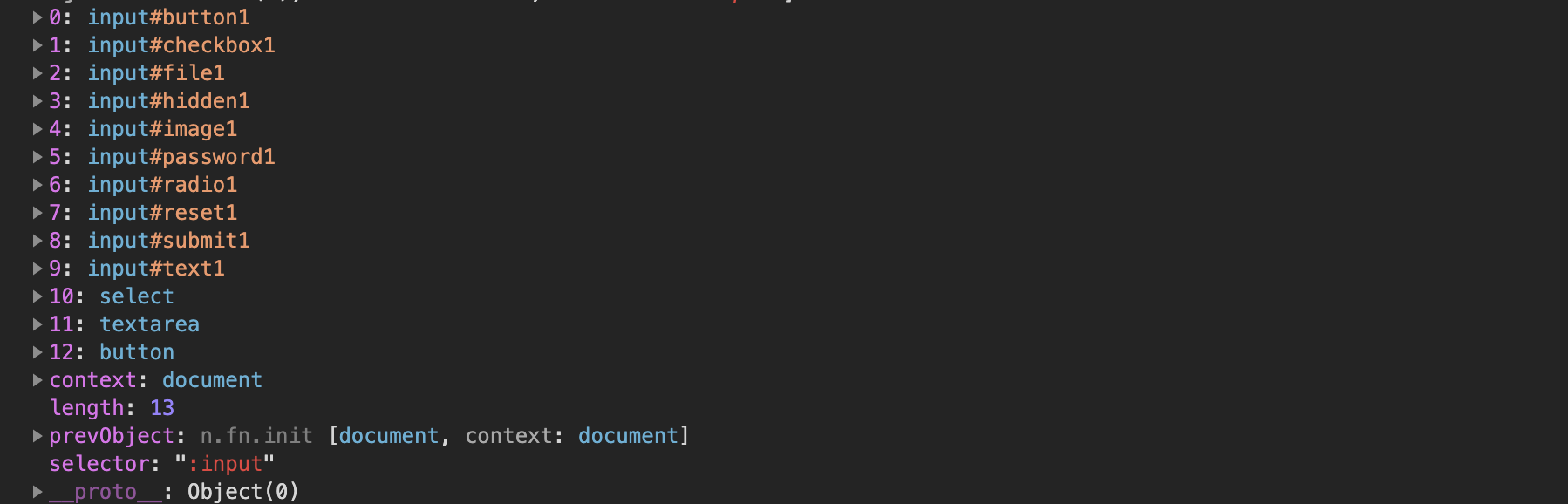##### 2、匹配所有的单行文本框

语法：$(":text") <form> <input type="button" value="Input Button" id="button1"/> <input type="checkbox" id="checkbox1"/> <input type="file" id="file1"/> <input type="hidden" id="hidden1"/> <input type="image" id="image1"/> <input type="password" id="password1"/> <input type="radio" id="radio1"/> <input type="reset" id="reset1"/> <input type="submit" id="submit1"/> <input type="text" id="text1"/> <select><option>Option</option></select> <textarea></textarea> <button>Button</button> </form> <script>$(function(){
console.log($(":text")); }) </script>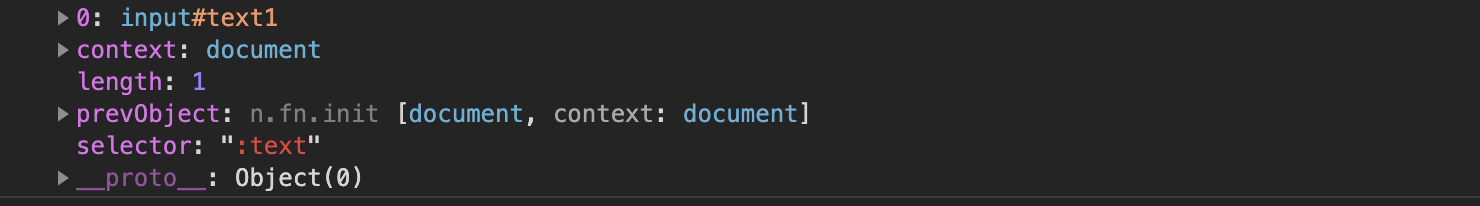##### 3、匹配所有密码框 语法：$(":password")

<form>
<input type="button" value="Input Button" id="button1"/>
<input type="checkbox" id="checkbox1"/>
<input type="file" id="file1"/>
<input type="hidden" id="hidden1"/>
<input type="image" id="image1"/>
<input type="reset" id="reset1"/>
<input type="submit" id="submit1"/>
<input type="text" id="text1"/>
<select><option>Option</option></select>
<textarea></textarea>
<button>Button</button>
</form>
<script>

$(function(){ console.log($(":password"));
})

</script>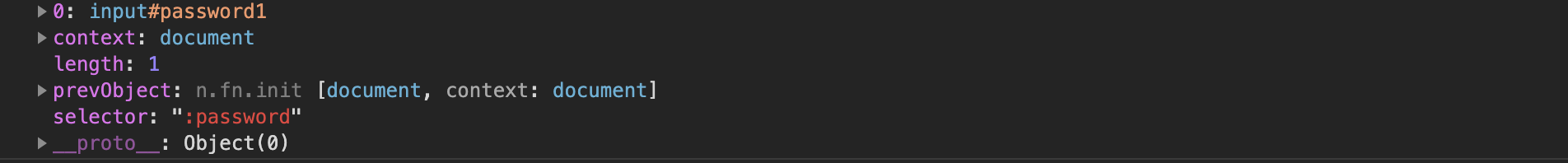##### 4、匹配所有单选按钮

语法：$(":radio") <form> <input type="button" value="Input Button" id="button1"/> <input type="checkbox" id="checkbox1"/> <input type="file" id="file1"/> <input type="hidden" id="hidden1"/> <input type="image" id="image1"/> <input type="password" id="password1"/> <input type="radio" id="radio1"/> <input type="reset" id="reset1"/> <input type="submit" id="submit1"/> <input type="text" id="text1"/> <select><option>Option</option></select> <textarea></textarea> <button>Button</button> </form> <script>$(function(){
console.log($(":radio")); }) </script>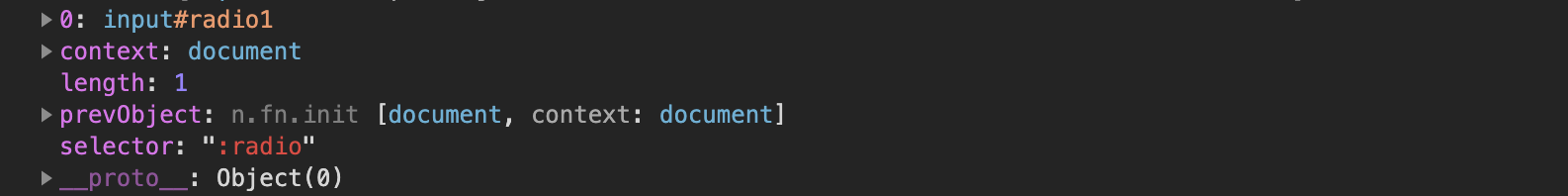##### 5、匹配所有复选框 语法：$(":checkbox")

<form>
<input type="button" value="Input Button" id="button1"/>
<input type="checkbox" id="checkbox1"/>
<input type="file" id="file1"/>
<input type="hidden" id="hidden1"/>
<input type="image" id="image1"/>
<input type="reset" id="reset1"/>
<input type="submit" id="submit1"/>
<input type="text" id="text1"/>
<select><option>Option</option></select>
<textarea></textarea>
<button>Button</button>
</form>
<script>

$(function(){ console.log($(":checkbox"));
})

</script>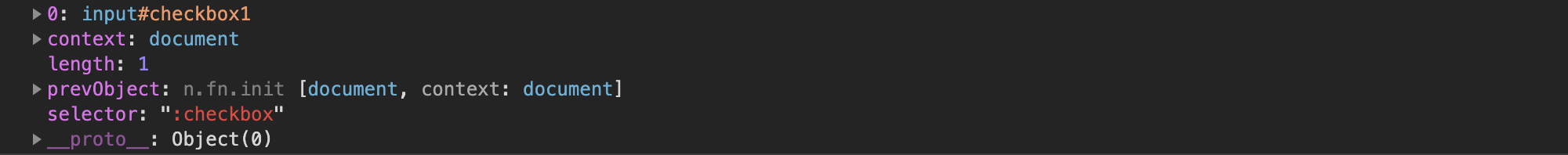##### 6、匹配所有提交按钮

语法：$(":submit") 理论上只匹配 type=“submit” 的input或者button，但是现在的很多浏览器，button元素默认的type即为submit，所以很多情况下，不设置type的button也会成为筛选结果。为了防止歧义或者误操作，建议所有的button在使用时都添加type属性。 <form> <input type="button" value="Input Button" id="button1"/> <input type="checkbox" id="checkbox1"/> <input type="file" id="file1"/> <input type="hidden" id="hidden1"/> <input type="image" id="image1"/> <input type="password" id="password1"/> <input type="radio" id="radio1"/> <input type="reset" id="reset1"/> <input type="submit" id="submit1"/> <input type="text" id="text1"/> <select><option>Option</option></select> <textarea></textarea> <button>Button</button> </form> <script>$(function(){
console.log($(":submit")); }) </script>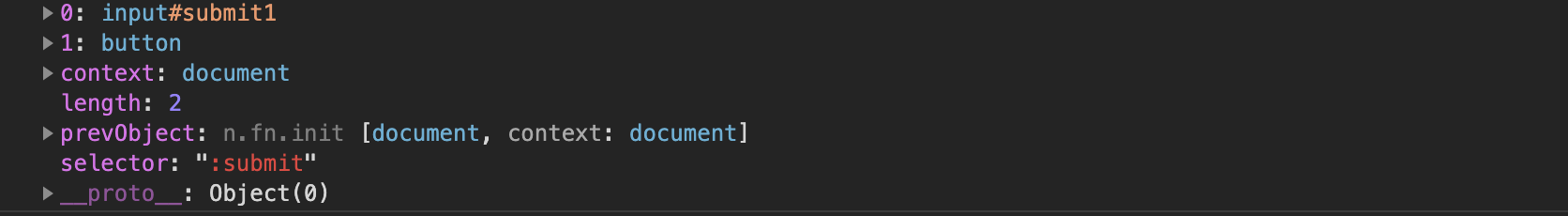##### 7、匹配所有图像域 语法：$(":image")

<form>
<input type="button" value="Input Button" id="button1"/>
<input type="checkbox" id="checkbox1"/>
<input type="file" id="file1"/>
<input type="hidden" id="hidden1"/>
<input type="image" id="image1"/>
<input type="reset" id="reset1"/>
<input type="submit" id="submit1"/>
<input type="text" id="text1"/>
<select><option>Option</option></select>
<textarea></textarea>
<button>Button</button>
<img src="" />
</form>
<script>

$(function(){ console.log($(":image"));
})

</script>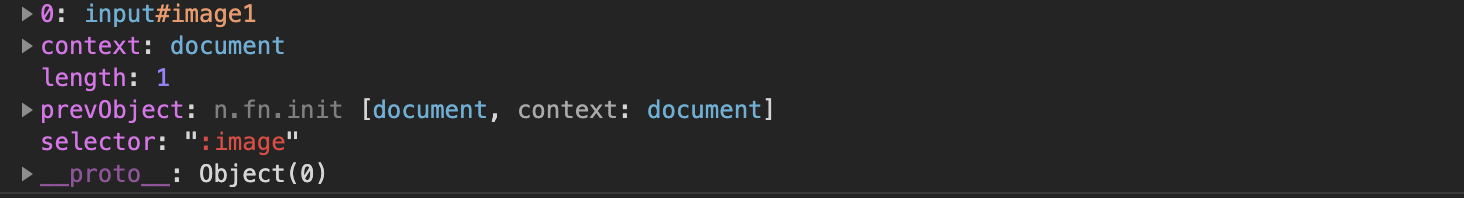##### 8、匹配所有重置按钮

语法：$(":reset") <form> <input type="button" value="Input Button" id="button1"/> <input type="checkbox" id="checkbox1"/> <input type="file" id="file1"/> <input type="hidden" id="hidden1"/> <input type="image" id="image1"/> <input type="password" id="password1"/> <input type="radio" id="radio1"/> <input type="reset" id="reset1"/> <input type="submit" id="submit1"/> <input type="text" id="text1"/> <select><option>Option</option></select> <textarea></textarea> <button>Button</button> <img src="" /> </form> <script>$(function(){
console.log($(":reset")); }) </script>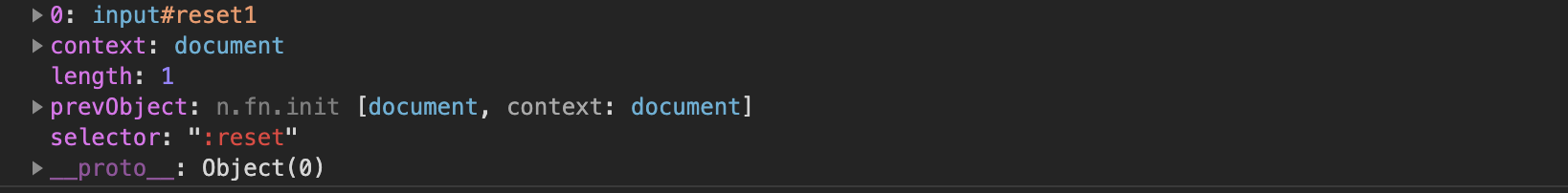##### 9、匹配所有按钮 语法：$(":button"）

<form>
<input type="button" value="Input Button" id="button1"/>
<input type="checkbox" id="checkbox1"/>
<input type="file" id="file1"/>
<input type="hidden" id="hidden1"/>
<input type="image" id="image1"/>
<input type="reset" id="reset1"/>
<input type="submit" id="submit1"/>
<input type="text" id="text1"/>
<select><option>Option</option></select>
<textarea></textarea>
<button>Button</button>
<img src="" />
</form>
<script>

$(function(){ console.log($(":button"));
})

</script>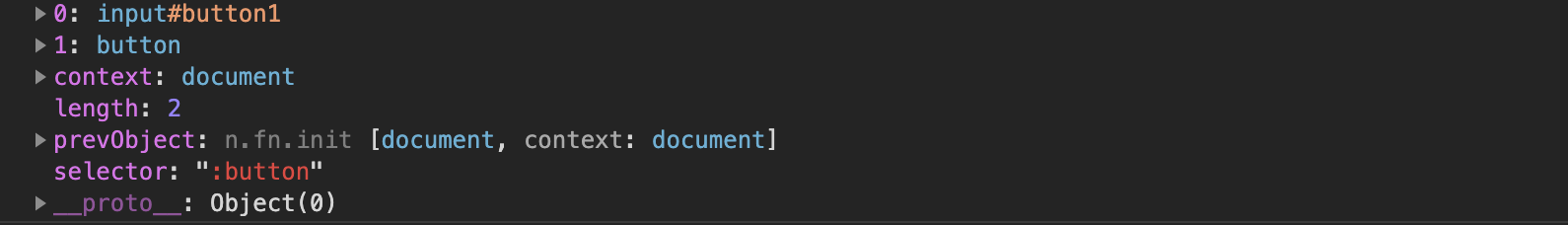##### 10、匹配所有文件域

语法：$(":file") <form> <input type="button" value="Input Button" id="button1"/> <input type="checkbox" id="checkbox1"/> <input type="file" id="file1"/> <input type="hidden" id="hidden1"/> <input type="image" id="image1"/> <input type="password" id="password1"/> <input type="radio" id="radio1"/> <input type="reset" id="reset1"/> <input type="submit" id="submit1"/> <input type="text" id="text1"/> <select><option>Option</option></select> <textarea></textarea> <button>Button</button> <img src="" /> </form> <script>$(function(){
console.log($(":file")); }) </script>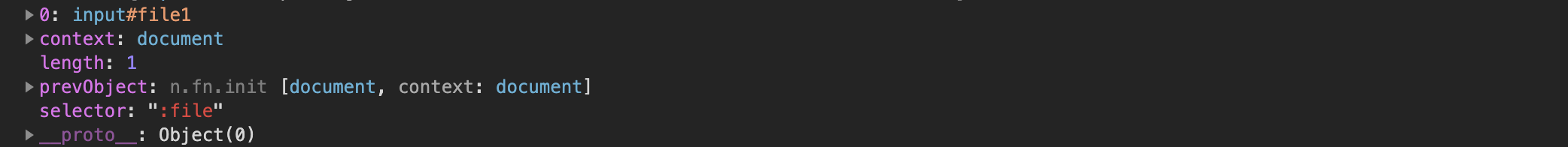#### 五、表单对象属性选择器 :enabled匹配所有可用元素 :disabled匹配所有不可用元素 :checked匹配所有选中的被选中元素(复选框、单选框等) :selected匹配所有选中的option元素(下拉列表) #### 六、可见性选择器 ##### 1、匹配所有不可见元素 语法：$(":hidden")

匹配所有不可见元素，或者type为hidden的元素

以下几种情况的元素是隐藏元素：注释：该选择器对 visibility:hidden 和 opacity: 0 的元素不起作用。

设置为 display:none
带有 type=“hidden” 的表单元素
width 和 height 设置为 0
隐藏的父元素（这也会隐藏子元素

语法：$(":visible") #### 七、子元素选择器 ##### 1、先位置，在元素 语法：$(" :first-child") 匹配第一个子元素
语法： $(":last-child") <ul> <p id="p1"></p> <li id="li1"><span></span></li> <li id="li2"></li> <li id="li3"></li> </ul> <script>$(function(){
console.log($("ul li:first-child")); //没有匹配到，因为ul到第一个子元素不是li }) </script>  ##### 2、先元素，在位置 语法：$(“E:first-of-type”)

语法：$(“E:last-of-type”) <ul> <p id="p1"></p> <li id="li1"><span></span></li> <li id="li2"></li> <li id="li3"></li> </ul> <script>$(function(){

##### 4、匹配给定的属性是以某些值开始的元素

语法：$（" [attribute^=value]"） <p class="fooof">第一个p</p> <p class="foww">第二个p</p> <p class="fooof">第三个p</p> <div id="box1">第一个div</div> <div id="box2">第二个div</div> <div id="box3">第三个div</div> <script>$(function(){
console.log($("[class^='f']")); }) </script>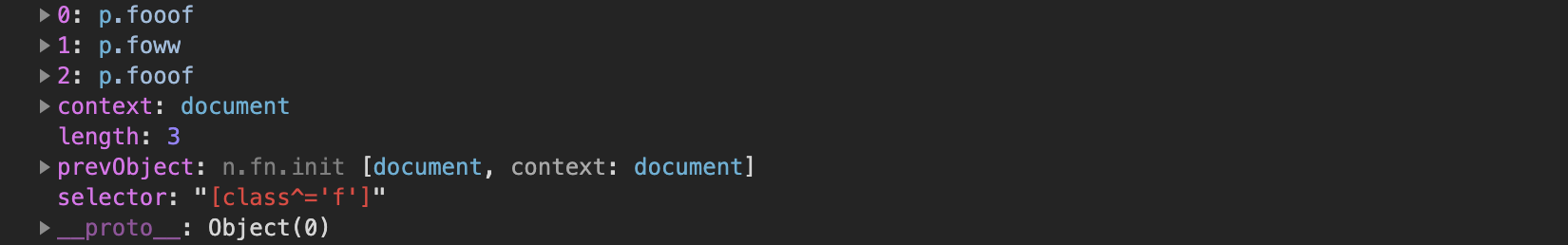##### 5、匹配给定的属性是以某些值结尾的元素 语法： （ " [ a t t r i b u t e （"[attribute =value] "） ##### 6、匹配给定的属性是以包含某些值的元素 语法：$（" [attribute*=value]"）

7、复合属性选择器，需要同时满足多个条件时使用。

语法：$（"[attrSel1][attrSel2][attrSelN]"） <p class="fooof">第一个p</p> <p class="foww">第二个p</p> <p class="fooof">第三个p</p> <div id="box1">第一个div</div> <div id="box2">第二个div</div> <div id="box3" class="box">第三个div</div> <script>$(function(){
console.log($("[class][id]")); }) </script>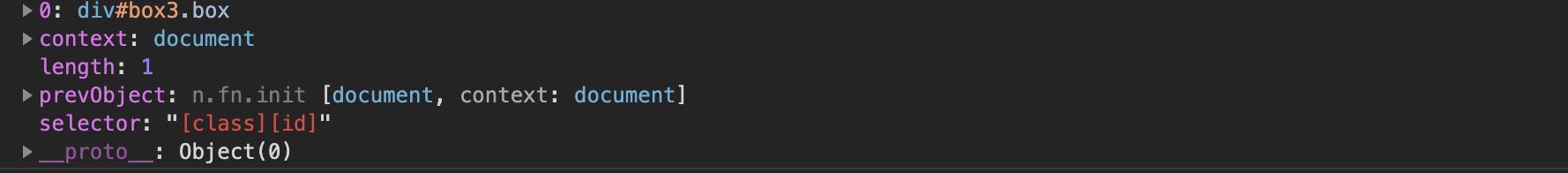#### 九、内容选择器 ##### 1、匹配包含给定文本的元素 语法：$(":contains(string)")

<p class="fooof">第一个p</p>
<p class="foww">第二个p</p>
<p class="fooof">第三个p</p>
<div id="box1">第一个div</div>
<div id="box2">第二个div</div>
<div id="box3" class="box">第三个div</div>
<script>
$(function(){ console.log($("body :contains('二')"));
})
</script>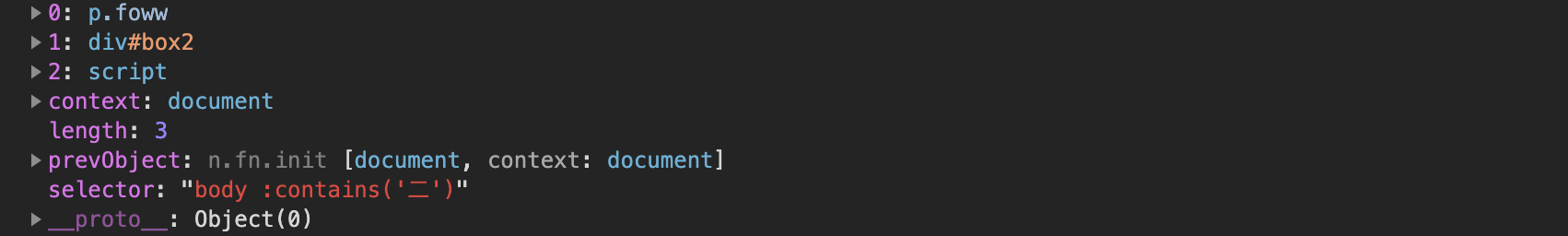语法：$(":empty") ##### 3、匹配含有选择器所匹配的元素的元素，参数selector是一个标签选择器 语法：$(":has(selector)")

<p class="fooof">第一个p</p>
<p class="foww"><span></span></p>
<p class="fooof">第三个p</p>
<div id="box1">第一个div</div>
<div id="box2"></div>
<div id="box3" class="box">第三个div</div>
<script>
$(function(){ console.log($("p:has(span)"));
})
</script>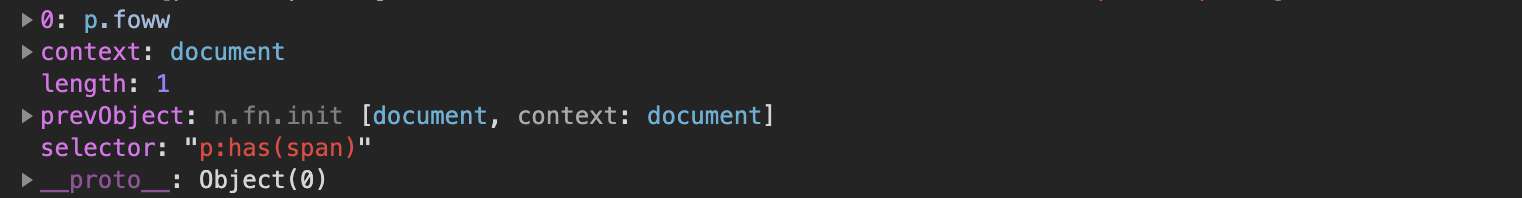##### 4、匹配含有子元素或者文本的元素

语法：$(":parent") <p class="fooof">第一个p</p> <p class="foww"></p> <p class="fooof">第三个p</p> <div id="box1">第一个div</div> <div id="box2"></div> <div id="box3" class="box">第三个div</div> <script>$(function(){
console.log($("body :parent")); }) </script>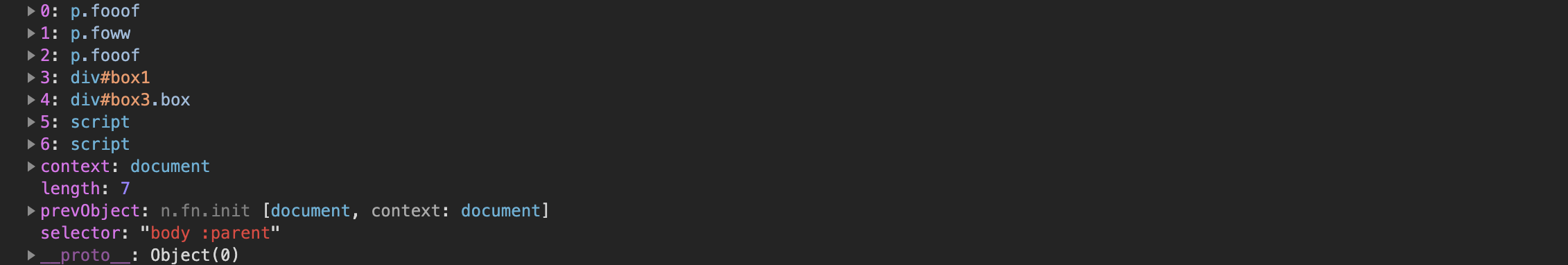展开全文• 1.jQuery获取表单的全部数据。 jQuery序列化表单数据 可以使用serialize()和 serializeArray()。 它们的区别如下： (1).serialize()方法 格式：var data=$("form").serialize(); 　功能：将表单内容序列化成一...

1.jQuery获取表单的全部数据。

jQuery序列化表单数据 可以使用serialize()和 serializeArray()。

它们的区别如下：

(1).serialize()方法

格式：var data= $("form").serialize(); 功能：将表单内容序列化成一个字符串。 这样在ajax提交表单数据时，就不用一一列举出每一个参数。只需将data参数设置为$("form").serialize() 即可。

(2).serializeArray()方法

格式：varjsonData = $("form").serializeArray(); 功能：将页面表单序列化成一个JSON结构的对象。注意不是JSON字符串。 比如，[{"name":"lihui","age":"20"},{...}] 获取数据为 jsonData.name data={"username":"kidd","mobile":"12312312312"} 2.jQuery获取表单指定name或者class或者id的数据值。 姓名<input type="text"id="username" class="username" name="username"value=""> 手机<input type="text" id="mobile"class="mobile" name="mobile" value=""> //获取姓名的数据$('#username').val();                      //id选择器
$('.username').val(); //class选择器$('[name="username"]').val();              //属性选择器
$('input:eq(0)').val(); //过滤选择器$('input:first').val();                    //过滤选择器
$(':text:eq(0)').val(); //表单选择器$(':text:first').val();                    //表单选择器 

//获取手机的数据
$('#mobile').val(); //id选择器$('.mobile').val();                      //class选择器
$('[name="mobile"]').val(); //属性选择器$('input:eq(1)').val();                  //过滤选择器
$('input:last').val(); //过滤选择器$(':text:eq(1)').val();                  //表单选择器
$(':text:last').val(); //表单选择器  3.jQuery如何修改表单指定name,class,id的值。 修改表单元素的值，$(选择器).val(newdata);

//修改姓名
$('#username').val(newdata); //id选择器$('.username').val(newdata);                      //class选择器
$('[name="username"]').val(newdata); //属性选择器 //修改电话$('#mobile').val(newdata);                        //id选择器
$('.mobile').val(newdata); //class选择器$('[name="mobile"]').val(newdata);                //属性选择器

4.jQuery如何找到父元素下指定class的子元素。

<div class="regist">
<divclass="name_element">
<label>姓名</label>
<inputtype="text" name="name">
<span></span>
</div>
<divclass="mobile_element">
<label>手机</label>
<inputtype="text" name="mobile">
<span></span>
</div>
</div>

想要获取到regist下面的姓名中input的值可以使用后代选择器或者查找函数。

后代选择器：alert($('.registdiv:eq(0) [name="name"]').val()); find函数： alert($('.regist').find('[name="name"]').val());

练习demo

<!DOCTYPE html>
<html lang="en">
<meta charset="UTF-8">
<title>jQuery表单选择器</title>
<style type="text/css">
.regist div{margin:10px auto;width: 500px;}
.regist label{display:inline-block;width:90px;text-align:right;}
.submit_element{width:100px;text-align: center;}
</style>
<script src="http://cdn.bootcss.com/jquery/3.1.1/jquery.min.js"></script>
<body>
<form action="demo161229.php" method="post" class="regist_form">
<div class="regist">
<div class="name_element">
<label>姓名</label>
<input type="text" name="name">
<span></span>
</div>
<label>密码</label>
<span></span>
</div>
<label>确认密码</label>
<span></span>
</div>
<div class="sex_element">
<label>性别</label>
<span></span>
</div>
<div class="submit_element">
<input type="button" class="regist_btn" value="注册">
</div>
</div>
</form>
</body>
<script type="text/javascript">
function checkName(){
var name=$.trim($('[name="name"]').val());
var msg=null;
var flag=false;
if(name.length==0){
msg='姓名不能为空';
}else if(name.length<3){
msg='姓名不能少于3个字';
}else{
msg='ok';
flag='true';
}
$('.name_element span').text(msg); return flag; } function checkPassword(){ var password=$('[name="password"]').val();
var msg=null;
var flag=false;
msg='密码不能为空';
msg='密码不能少于6个字符';
}else{
msg='ok';
flag='true';
}
$('.password_element span').text(msg); return flag; } function checkRepassword(){ var password=$('[name="password"]').val();
var repassword=$('[name="repassword"]').val(); var msg=null; var flag=false; if(repassword.length==0){ msg='确认密码不能为空'; }else if(password!=repassword){ msg='两次密码不一致'; }else{ msg='ok'; flag='true'; }$('.repassword_element span').text(msg);
return flag;
}
$(function(){$('[name="name"]').blur(function(){
checkName();
});
$('[name="password"]').blur(function(){ checkPassword(); });$('[name="repassword"]').blur(function(){
});
$('.regist_btn').click(function(){ if(!checkName()){ return; } if(!checkPassword()){ return; } if(!checkRepassword()){ return; } var url=$('form').attr('action');
//序列化表单提交
// $.post(url,$('form').serializeArray(),function(res){
// 	res=$.parseJSON(res); // alert(res.info); // }); //一个一个表单元素提交 var name=$.trim($('[name="name"]').val()); var password=$('[name="password"]').val();
var repassword=$('[name="repassword"]').val(); var sex=$('[name="sex"]').val();
$.post(url,{name:name,password:password,repassword:repassword,sex:sex},function(res){ res=$.parseJSON(res);
});
});
});
</script>
</html>


后台页面

<?php
$name=$_POST['name'];
$mobile=$_POST['mobile'];
$sex=$_POST['sex'];
$time=time(); if($sex==1){
$sex='先生'; }else{$sex='女士';
}
$time=date('Y-m-d H:i:s',$time);
$info='恭喜你,'.$name.$sex.'你在'.$time.'成为了我们的会员！';
$res=array( "status"=>1, "info"=>$info
);
echo json_encode($res); die(); ?> 展开全文• 主要介绍了jQuery基本选择器标签选择器的相关资料,本文介绍的非常详细，具有参考借鉴价值，需要的朋友可以参考下 • 去年我学jQuery的时候，曾经做过一点选择器（selector）的笔记。 这几天拿出来看了一下，发现很多都...标签选择器，匹配所有使用E标签的元素 3. .info class选择器，匹配所有class属性中包含info的元素 4. #footer • prototype: var element =$(‘eleId’) jquery: var element = $(‘#eleId’) DOM: var element = document.getElementById(‘eleId’) 以上三种选择方式是等价的,相比prototype来说jquery多了个#号 ... • 表单选择器 1. :button Selector 1. jQuery(“:button”) 2. 选择所有元素和类型为按钮的元素 2. :checkbox Selector 1. jQuery(“:checkbox”) 2. 选择所有元素和类型为复选框的元素 3. :checked ... • 1. 标签选择器（元素选择器） 语法：$("html标签名") 获得所有匹配标签名称的元素 案例： $("div") 选择元素名为<div>的所有元素 2. id选择器 语法：$("#id的属性值") 获得与指定id属性值匹配的元素 ...

### 文章目录

选择器：筛选具有相似特征的元素(标签)

# 1. 基本选择器

1. 标签选择器（元素选择器）
语法： $("html标签名") 获得所有匹配标签名称的元素 案例：$("div") 选择元素名为<div>的所有元素
2. id选择器
语法： $("#id的属性值") 获得与指定id属性值匹配的元素 案例：$("#one") 选择 id 为 one 的元素
3. 类选择器
语法： $(".class的属性值") 获得与指定的class属性值匹配的元素 案例：$(".mini") 选择class 为 mini 的所有元素
4. 并集选择器：
语法： $("选择器1,选择器2....") 获取多个选择器选中的所有元素 案例：$("span,#two") 选择所有的 <span> 元素和 id 为 two 的元素


# 2. 层级选择器

1. 后代选择器
语法： $("A B ") 选择A元素内部的所有B元素 案例：$("body div")	选择<body> 内所有 <div>元素,支持多级
2. 子选择器
语法： $("A > B") 选择A元素内部的所有B子元素 案例：$("body > div") 选择<body> 内子 <div>,不支持多级
3. 相邻元素选择器
语法： $("A + B") 案例：$("#one+div") 选择 id 为 one 的下一个 div 元素
4. 兄弟元素选择器
语法： $("A ~ B") 匹配 A 元素之后的所有 B 元素 案例：$("#two~div") 选择 id 为 two 的元素后面的所有 div 兄弟元素


# 3. 属性选择器

[attribute] 匹配包含给定属性的元素。
$("div[title]") 含有属性title 的div元素 [attribute=value] 匹配给定的属性是某个特定值的元素$("div[title='test']")	属性title值等于test的div元素

[attribute!=value] 匹配所有不含有指定的属性，或者属性不等于特定值的元素。
$("div[title!='test']") 属性title值不等于test的div元素(没有属性title的也将被选中) [attribute^=value] 匹配给定的属性是以某些值开始的元素$("div[title^='te']")	属性title值 以te开始 的div元素
$(":text[name^=tel]") 选中name以tel开头的输入框 [attribute$=value] 匹配给定的属性是以某些值结尾的元素
$("div[title$='est']")	属性title值 以est结束 的div元素

[attribute*=value] 匹配给定的属性是以包含某些值的元素
$("div[title*='es']") 属性title值 含有es的div元素 [attrSel1][attrSel2][attrSelN] 复合属性选择器，需要同时满足多个条件时使用$("div[id][title*='es']") 选取有属性id的div元素，然后在结果中选取属性title值含有“es”的 div 元素



# 4. 基本过滤器

1. 首元素选择器
语法： :first 获得选择的元素中的第一个元素
案例： $("div:first") 第一个 div 元素 2. 尾元素选择器 语法： :last 获得选择的元素中的最后一个元素 案例：$("div:last") 最后一个 div 元素

3. 非元素选择器
语法： :not(selector) 不包括指定内容的元素
案例： $("div:not(.one)") class不为 one 的所有 div 元素$("tr:not(:first)") 选tr元素，但排除第一个

4. 偶数选择器
语法： :even 偶数，从 0 开始计数
案例： $("div:even") 索引值为偶数的 div 元素 5. 奇数选择器 语法： :odd 奇数，从 0 开始计数 案例：$("div:odd") 索引值为奇数的 div 元素

6. 等于索引选择器
语法： :eq(index) 指定索引元素
案例： $("div:eq(3)") 索引值为等于 3 的 div 元素 7. 大于索引选择器 语法： :gt(index) 大于指定索引元素 案例：$("div:gt(3)") 索引值为大于 3 的 div 元素

8. 小于索引选择器
语法： :lt(index) 小于指定索引元素
案例： $("div:lt(3)") 索引值为小于 3 的 div 元素 9. 标题选择器 语法： :header 获得标题（h1~h6）元素，固定写法 案例：$(":header") 所有的标题元素

10. 动画选择器
语法： :animated  匹配所有正在执行动画效果的元素
案例： $(":animated")  # 5. 内容过滤器 :contains(text) 匹配包含给定文本的元素$("div:contains('di')") 选择 含有文本 'di' 的 div 元素

:empty 匹配所有不包含子元素或者文本的空元素
$("div:empty") 选择不包含子元素(或者文本元素) 的 div 空元素 :parent 匹配含有子元素或者文本的元素$("div:parent")	选择含有子元素(或者文本元素)的div元素

:has(selector) 匹配含有选择器所匹配的元素的元素
$("div:has(.mini)") 选择含有 class 为 mini 元素的 div 元素  # 6. 表单过滤器: :input 匹配所有 input, textarea, select 和 button 元素 :text 匹配所有 文本输入框 :password 匹配所有的密码输入框 :radio 匹配所有的单选框 :checkbox 匹配所有的复选框 :submit 匹配所有提交按钮 :image 匹配所有 img 标签 :reset 匹配所有重置按钮 :button 匹配所有 input type=button <button>按钮 :file 匹配所有 input type=file 文件上传 :hidden 匹配所有不可见元素 display:none 或 input type=hidden  # 7. 表单对象属性过滤器 1. 可用元素选择器 语法： :enabled 获得可用元素 案例：$("input[type='text']:enabled") | $(":text:enabled") 表单内 可用的input 2. 不可用元素选择器 语法： :disabled 获得不可用元素 案例：$(":text:disabled") 表单内 不可用input
3. 选中选择器
语法： :checked 获得单选/复选框选中的元素
案例： $("input[type='checkbox']:checked").length |$(":checkbox:checked").length
获取多选框选中的个数 利用 jQuery 对象的 length 属性获取复选框选中的个数
==================================================================
// 获取全部选中的复选框标签对象
var $checkboies =$(":checkbox:checked");
// 老式遍历
// for (var i = 0; i < $checkboies.length; i++){ // alert($checkboies[i].value );
// }

// each方法是jQuery对象提供用来遍历元素的方法
// 在遍历的function函数中，有一个this对象，这个this对象，就是当前遍历到的dom对象
$checkboies.each(function () { alert( this.value ); }); 4. 选中选择器 语法： :selected 获得下拉框选中的元素 案例： <select id="test" name=""> <option value="1">text1</option> <option value="2">text2</option> </select> 获取选中的option标签对象 var options =$("select option:selected");
options.val() 拿到选中项的 value 值	(1)
options.text() 拿到选中项的 文本值	(text1)


# 8. jQuery 元素筛选

eq() 获取给定索引的元素 	功能跟 :eq() 一样
first() 获取第一个元素 	功能跟 :first 一样
last() 获取最后一个元素 	功能跟 :last 一样
filter(exp) 留下匹配的元素
is(exp) 判断是否匹配给定的选择器，只要有一个匹配就返回，true
has(exp) 返回包含有匹配选择器的元素的元素 功能跟 :has 一样
not(exp) 删除匹配选择器的元素 功能跟 :not 一样
children(exp) 返回匹配给定选择器的子元素 	功能跟 parent>child 一样
find(exp) 返回匹配给定选择器的后代元素 		功能跟 ancestor descendant 一样
next() 返回当前元素的下一个兄弟元素 			功能跟 prev + next 功能一样
nextAll() 返回当前元素后面所有的兄弟元素 	功能跟 prev ~ siblings 功能一样
nextUntil() 返回当前元素到指定匹配的元素为止的后面元素
parent() 返回父元素
prev(exp) 返回当前元素的上一个兄弟元素
prevAll() 返回当前元素前面所有的兄弟元素
prevUnit(exp) 返回当前元素到指定匹配的元素为止的前面元素
siblings(exp) 返回所有兄弟元素
$(this).siblings("li") 获取当前 li 元素所有兄弟元素 add() 把 add 匹配的选择器的元素添加到当前 jquery 对象中  展开全文• jQuery允许开发者使用从CSS1到CSS3... jQuery选择器类型 jQuery选择器主要分为四类： 1、基本选择器 2、层次选择器 3、过滤选择器 4、表单选择器 由于过滤选择器内容比较多，因此本文仅介绍前两种，下篇文章将介绍后两 • jquery选择器中的通配符经常用到，大致的用法总结如下：$("input[id^='code']");//id属性以code开始的所有input标签 $("input[id$='code']");//id属性以code结束的所有input标签 $("input[id*='code']");//id属性... • 1.标签选择器：$(“element”) 其中，参数element，表示待查找的HTML标记名，如$(“div”),标签选择器获取元素的方式是高效的，因为它继承自javascript中的getEmelentsByTagName,它获取的是整个元素的集合。 2.ID... • jQuery选择器可谓之强大无比，这里简单地总结一下常用的元素查找方法$(“#myELement”) 选择id值等于myElement的元素，id值不能重复在文档中只能有一个id值是myElement所以得到的是唯一的元素 $(“div”) 选择... • 吐血整理，如有错误，欢迎指出，感激不尽~ 目录1. jq选择器2. 属性操作3. Class操作4. 样式操作5. 内容操作6....1. jq选择器 jq的选择器，就是在执行jq函数时传参 ...标签选择器:$(span); 通配符选择器: $(* • 1.jQuery选择器 1.1jQuery基础选择器 原生js获取元素方式很多，很杂，而且兼容性情况不一致，因此jQuery给我们做了封装，使获取元素统一标准。$(‘选择器’) //里面选择器直接写css选择器即可，但是要加引号 原创...
• 基本选择器jQuery中最常用的选择器，也是最简单的选择器，它主要是通过元素的id、class、标签等来查找HTML中的DOM元素。在网页中每个id只能使用一次，class允许重复使用。在jQuery应用中，可以使用这些基本选择器...
• jquery中只有三种选择器 二、基本选择器 1、定位条件 可以根据ID编号，根据标签类型名，根据Class名(和CSS一样都是三种) 2、选择器的使用(第四种几乎不用) $("#idname") 代替document.getElementById(“idname”)... • jQuery使用了一套css选择器，共有5种，即标签选择器，ID选择器，类选择器，通用选择器和群组选择器，现分述如下： 1. 标签选择器 用于选择HTML页面中已有的标签元素，又称元素选择器，格式：$(“element”)，如$(... • 标签选择器】 <script src="https://code.jquery.com/jquery-3.4.0.min.js"></script> <!-- 标签选择器 --> <h1>清平调</h1> <h3>李白</h3> <script>$...javascript
• 我正在阅读使用jQuery选择器使用通配符或正则表达式（不确定的确切术语）的文档。 I have looked for this myself but have been unable to find information on the syntax and how to use it. 我自己一直在寻找，...javascript regex
• 选择器并没有一个固定的定义，在某种程度上说，jQuery选择器和样式表中的选择器十分相似。选择器具有如下特点： 1．简化代码的编写 2．隐式迭代 3．无须判断对象是否存在 jQuery选择器可谓之强大无比，这里简单...
• ## JQuery选择器

千次阅读 2021-02-01 06:52:20
选择下标为0开始 到 m之前结束(不包括m)的元素 ) $('#one').children('li:first').css( ) ( 给元素的指定子元素添加样式 )$('.one').parent( ).css( ) ( 给元素的父元素添加样式 ) $('.one').parents( ).css( ) ( ... • 本文为大家分享了jQuery常用选择器的具体代码，供大家参考，具体内容如下 1、jQuery：（使用jQuery一定标明我们使用的版本号） 它是一个使用原生的JS来封装的常用的方法的类库（解决了浏览器的兼容问题） 2、... • jquery系列教程1-选择器全解jquery基本选择器jquery基本选择器，包括id选择器、class选择器、标签选择器、通配符选择器，同时配合选择器的空格、逗号等语法，可以实现大部分需要的选择功能。 代码如下：$("#myid");...class id
• $的选择器部分: 凡是运用$,其返回值是一个object $选择器主要用于选择标签.基本用法是同css的选择器.但是,很让人兴奋的是,他支持常见的浏览器,而css中很多选择器是IE6所不支持的. 1.基本选择器(3种): —-凡是运用$,...
• 基本选择器JQuery中最常用的选择器，也是最简单的选择器，它通过元素id、class 和标签名来查找DOM元素。这个非常重要，下面的内容都是以此为基础，逐级提高的。 1).“\$(“#id”)”，获取id指定的元素，id是全局...
• 想要使用jQuery选择器选择多个元素，要怎么实现？下面本篇文章就来给大家介绍jQuery选择器选择多个元素的方法，希望对大家有所帮助。在jQuery中，可以使用以逗号(，)分隔的元素选择器列表--多个元素选择器，或*选择...
• jQuery选择器使得获得页面元素变得更加容易、更加灵活，从而大大减轻了开发人员的压力。如同盖楼一样，没有砖瓦，就盖不起楼房。得不到元素谈何其他各种操作呢？可见,jQuery选择器的重要性。当然想一下子掌握所有...
• 本文实例讲述了JQuery标签页效果实现方法。分享给大家供大家参考，具体如下： 第一个标签页中鼠标滑过显示不同的标签页，第二个标签页中点击不同标签加载其他页面中的内容，加载等待的图片缓慢隐藏，效果图如下： /...
• 基本选择器jQuery中最常用的选择器，也是最简单的选择器，它通过元素id、class和标签名等来查找DOM元素。在网页中，每个id名称只能使用一次，class允许重复使用。  选择器  描述 返回  示例 #id 根据给定......